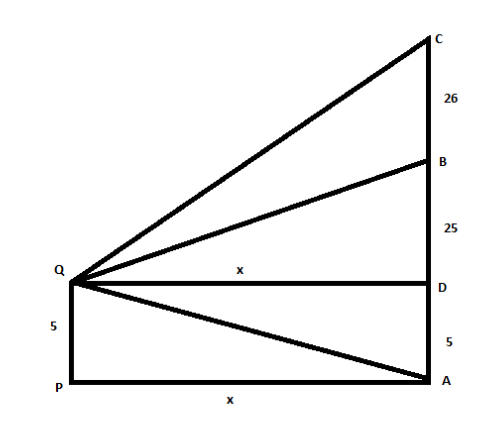QuestionAnswers

# A tower is $51$m high and has a mark at a height of $25$ m from the ground. Find at what distance the two parts subtend equal angles to an eye at the height of $5$m, from the ground.Verified
129.6k+ views
Hint: At first, we will consider the diagram given below. Then by using Pythagoras theorem we can find the solution of the given problem.It is given that the height of the tower is $51$m. It has a mark at a height of $25$m from the ground. We have to find the distance where the two parts subtend equal angles to an eye at the height of $5$m, from the ground.
Let us consider the diagram where,$AC = 51$, $B$ is the marked point such that, $AB = 25$. Again, $PQ = AD = 5$
It is given that,
$\angle CQB = \angle AQB$.
Therefore $QB$ is bisector of angle $\angle AQC$ and as such it divides the base $AC$ in the ratio of the arm of the angle
$\dfrac{{AB}}{{BC}} = \dfrac{{QA}}{{QC}}$
We are going to use Pythagoras theorem for following triangles,
From $\Delta QDA$ we have, $\sqrt {Q{D^2} + A{D^2}} = QA$
From $\Delta QDC$ we have, $\sqrt {Q{D^2} + D{C^2}} = QC$
Let us substitute these values of the sides in the above equation we get,
$\dfrac{{AB}}{{BC}} = \dfrac{{\sqrt {Q{D^2} + D{A^2}} }}{{\sqrt {Q{D^2} + D{C^2}} }}$
Let us consider, $PA = QD = x$
Let substitute these known as values we get,
$\dfrac{{25}}{{26}} = \dfrac{{\sqrt {{x^2} + {5^2}} }}{{\sqrt {{x^2} + {{46}^2}} }}$
On squaring both sides and cross multiplying we get,
$625({x^2} + {46^2}) = 676({x^2} + 25)$
Om simplifying the above equation, we get,
$51{x^2} = (625 \times {46^2} - 676 \times 25)$
Simplifying again we get,
${x^2} = 25600$
Taking square root on both sides of the above equation we get,
$x = 160$
Hence, the required distance is $160$m.

Note: Pythagoras theorem states that, for a right-angle triangle, the square of the hypotenuse is equal to the sum of the square of base and the square of perpendicular.
To find the value of x diagram plays a major role, it will help us by leading it to find the triangle to which the Pythagoras theorem is applied.